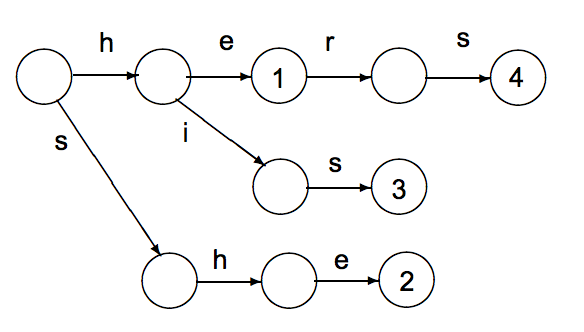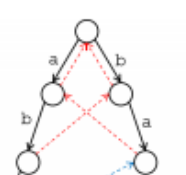## 開始介紹 Aho–Corasick Algorithm (AC Algorithm)

### 為何需要 AC Algorithm?

( 題外話: 參考這篇可以在 Jekyll 裡面加上 Latex )

### 透過 KMP 如果要處理多個搜尋字串的時間複雜度

\begin{align*} ((| P_1| )+ m) + ((| P_2| )+ m) + .... ((| P_k| )+ m) &= \sum_{i=1}^k | Pi | + k*m \end{align*}

### 建立字典樹### 關於字典樹的錯誤索引

KMP Fail Index (Function) :

• 透過單個搜尋字串建立
• 建立每個字元的錯誤索引 ( fail index )也就是比對到該字元如果發生錯誤，需要退到哪個 index 繼續之後的比對．
• Fail Index 在 KMP 中特別注意到連鎖字元 也就是 ABCEABC 其中的 ABC 就被認為是連鎖字元． 會直接尋找該字元前面的錯誤索引 (Fail Index) 避免在比對的時候多餘的移動． （這也是 KMP 勝過 MP 的重點)

ACA Fail Index :

• 針對處理多個字串
• 專注在多個字串重複出現的字元，不專注在連鎖字元• 將 root node 放入 node list ，並將移動的目標節點 移動到 node list 的第一個 (也就是 Root)
• 目標節點往 $a_l$ 走，該點的 fail index 為計算方式為:
• Parent node 的 fail index 是否有相當的位置 a
• 如果 Parent node 是 root ，則該點的 fail index 為 root
• 目標節點往 $b_r$ (這邊是採取 BFS 走法) ，方法跟 $a_l$\$ 一樣，結果 fail index 也是 root
• 目標節點 移動到 node list 的第二個 (也就是 $a_l$ )
• 目標節點 移動往 $b_l$ 移動，該點的 fail index 計算方式，如下:
• 由 $a_l$ 的 fail index 也就是 root 來看是否有 b 的路徑 ( 有的！ 就是 $b_r$ (2) )
• 所以 $b_l$ 的 fail index 記錄為 2 ，也就是說當他往下比對發生錯誤的時候，會移動到 $b_r$ (2) 繼續比對．
• 為了確保大家了解，再做一個，將目標節點 移動到 node list 的第三個 (也就是 $b_r$ ) ，並且往 $a_r$ 移動． 他的 fail index 計算如下:
• 由 parent $b_r$ 的 fail index （也就是 root ) 往下看是否有 a
• 有找到由 root 出發的 a 也就是 $a_l$ (1)
• 將 $a_r$ 的 fail index 記錄為 1

### AC Automation 的查詢• 挑出第一個字 haystack = a，從節點 root (0) 開始，沒有a 的路徑，往下
• haystack = s，(0) 有 s 的路徑，往節點 (3) 走
• haystack = h，(3) 有 h 的路徑，往節點 (4) 走
• haystack = e，(4) 沒有 e的路徑，往 fail index (1) 走，並且走到 (2)
• haystack = r，(2) 有 r 的路徑，往節點 (8) 走
• haystack = s，(8) 有 s 的路徑，往節點 (9) 走，並且找到整串字串 hers
• haystack = a, (9) 沒有 a 往 (0) 走

## 心得

AC Algorithm 其實寫程式的部分並不難．如同所有樹狀結構的演算法一樣，如何能夠清楚地呈現，並且能夠清楚的除錯才是困難的．